## CS 112 Lab 10: Shared-Memory Parallelism Using OpenMP

### Objectives:

1. Learn about parallel programming for multicore CPUs.
2. Learn about simple shared-memory parallelism.
3. Learn basic OpenMP pragmas.

### Introduction

Before 2005, CPU clock speeds rose dramatically, doubling roughly every two years. The following chart shows this change in speed over 40 years: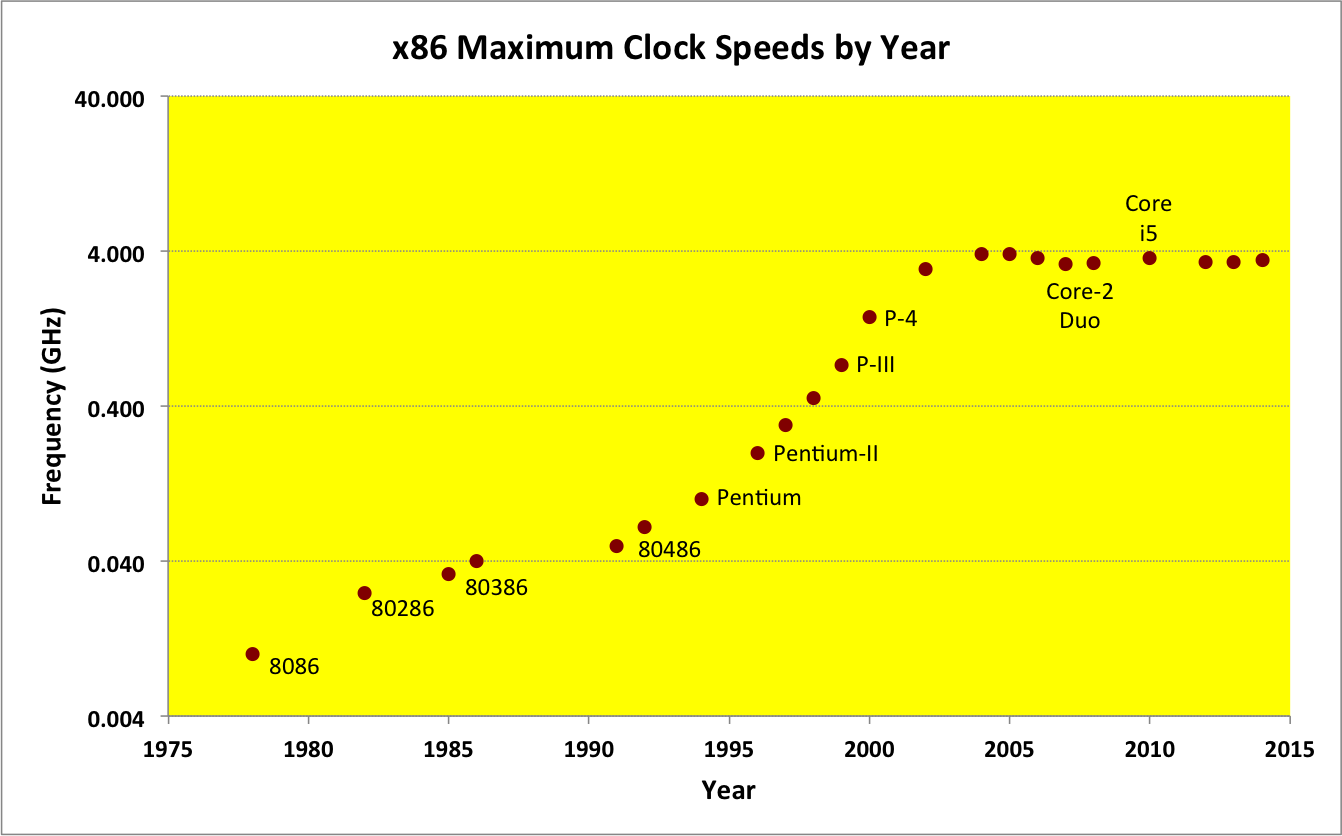Note that the scale on the left is a logarithmic scale, with each line being an order of magnitude increase.

Thanks to this rapid increase between 1975 and 2005, software developers and companies whose programs were sluggish and inefficient could count on faster clockspeeds in the future to make their programs perform acceptably for their users. In some circles, this time became known as the era of the free lunch for software developers, because the same software application would run twice as fast every two years, without its developers having to lift a finger.

As can be seen in the chart above, this "free lunch" era ended in 2005, when the costs of dealing with heat buildup, electron leakage, and other problems made it impractical for CPU manufacturers to keep increasing their clock speeds.

However, while clock speeds stopped increasing in 2005, Moore's Law has continued unabated: Every two years, CPU manufacturers have continued to squeeze the same amount of circuitry into half the area. In 2006, CPU manufacturers used this capability to fit two complete CPUs into the space formerly required for one, and called the results dual-core CPUs. By 2008, manufacturers could fit four CPUs into the space of one, yielding quad-core CPUs. By 2010, eight-core CPUs were available. And so on, with many-core CPUs available today: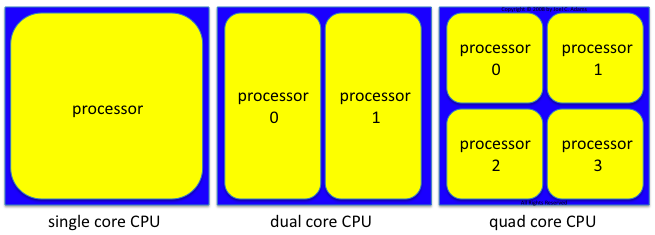In today's multicore world, CPUs are no longer speeding up, but a CPU's total processing capability continues to increase exponentially. That is, instead of a 5 GHz CPU, you can buy a 2.5 GHz dual-core CPU, whose two cores provide 5 GHz (2 x 2.5 GHz) worth of total CPU cycles. Instead of a 10 GHz CPU, you can buy a 2.5 GHz quad-core CPU, whose four cores provides 10 GHz (4 x 2.5 GHz) worth of total CPU cycles. Instead of a 20 GHz CPU, you can by a 2.5 GHz eight-core CPU, whose eight cores provide 20 GHz (8 x 2.5 GHz) worth of total CPU cycles. And so on. The challenge is now writing software that can take advantage of the multiple cores in these new CPUs.

The exercises we have done previously have all involved writing sequential programs, meaning the program's statements are performed one after another (i.e., in a sequence). This was the logical way to write software back when a CPU had just one core, since that one core could only perform one statement at a time.

By contrast, each core of a multicore CPU can be performing a different statement at the same time. This makes it possible to write parallel programs -- programs that are divided into pieces, with each piece running on a different core. This parallel execution can provide faster execution, but only if we redesign our software to: (i) break a problem into pieces, and (ii) have each core solve a different piece of the problem. This can make designing and debugging programs more difficult, because when multiple things can happen at the same time, the program logic has to be correct regardless of which combination of statements are performed simultaneously.

### Image Processing

Moore's Law has also affected other digital technologies, such as the image sensors used in digital cameras, camcorders, and smart phones. Thanks to Moore's Law, today's devices can take larger pictures (measured in megapixels) than their predecessors. For example, when digital cameras took "small" photos (e.g., 1-2 megapixels), sequential programs were sufficient to process the images, but as photos get larger (e.g., 20, 40, 80, 120, ... megapixels), processing those images sequentially may take too much time. And since the era of the "free lunch" is over, we cannot count on faster CPUs to speed up this processing. However, we can speed up the processing of large images by using the cores of our computer to process different parts of that image in parallel.

This week's exercise is to speed up an image-processing operation and explore how the parallelization works. We will start by timing the sequential version of an operation. Then we will convert that operation to a parallel version that will run faster on a multicore CPU, and time that parallel version using different numbers of cores. Finally, we will explore different ways such an operation can be "parallelized".

### RGB Colors

One way that one picture element or pixel of an image can be represented is as an RGB (Red, Green, Blue) color. For our purposes today, an RGB color is a triplet, each component of which has a value from 0 to 255. The first value in the triplet (R) represents the pixel's Red intensity, the second value (G) represents the pixel's Green intensity, and the third value (B) represents the pixel's Blue intensity. Each pixel's color is determined by its combination of these R, G, and B values, and each distinct combination of the three values produces a unique color. For example:

• (0, 0, 0) is black (The intensities of R, G, and B are all zero).
• (255, 0, 0) is red (R is at maximum intensity; G and B are at 0).
• (0, 255, 0) is green (R is at intensity 0; G is at maximum; B is at 0).
• (0, 0, 255) is blue (R and G are at intensity 0; B is at maximum).
• (255, 0, 255) is magenta (R and B are at maximum intensity; G is at 0).
• (255, 255, 0) is yellow (R and G are at maximum intensity; B is at 0).
• (0, 255, 255) is cyan (R is at intensity 0; G and B are at maximum).
• (255, 255, 255) is white (R, G, and B are all at maximum intensity).
It is also worth mentioning that any pixel whose three RGB values are the same will appear as some shade of gray.

Using this approach, a pixel can be set to any of 256 x 256 x 256 = 16,777,216 different colors, including 256 different shades of gray.

(For the sake of completeness, most pixels today also have a fourth component called the alpha value, denoted by the letter A. A pixel's alpha value controls its opacity: if a pixel's A-value is 0, it is completely transparent; if its A-value is 255, it is completely opaque; values in between 0 and 255 make pixels translucent. We will not use our pixels' A-values in today's exercise, but they are there, if you want to experiment with them in this week's project.)

### Processing an Image

Some common image-processing operations include: color inversion, color to black and white conversion, color to gray scale conversion, color to sepia conversion, image flipping (horizontal or vertical), image rotation (left or right), red eye reduction, line detection, and others.

Many of these operations use a similar approach, which we might describe with the following sequential pseudocode algorithm:

```    Canvas canvas1;
...
Canvas canvas2;
for each row r in canvas2 {
for each column c in canvas0 {
// using the pixel ar row r, column c on canvas1,
// do something with the pixel at row r, column c on canvas2
}
}
```
The operations main differences lie in what they do with the pixel, inside the inner loop.

It should be evident that the vast majority of the time required by one of these operations will be spent in the nested for loops. Keep that in mind as we proceed.

### Images and Memory Accesses

For simplicity's sake, suppose that we are using the preceding nested loops to process a 4x5 = 20 pixel image. We might visualize this image as follows, where each pi represents one of the image's pixels:We see this image as a 2-dimensional structure with 4 rows and 5 columns, and the nested loops will process the pixels row-by-row, as shown by the blue line below: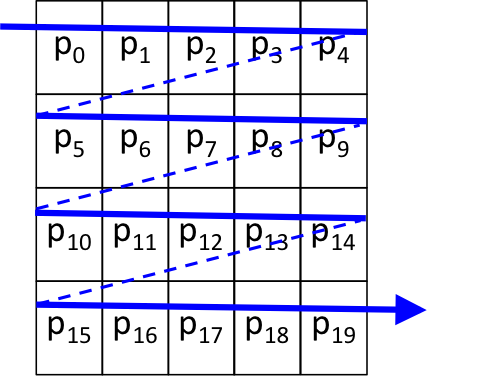However, since the image's pixels are stored in memory row-by-row, and the computer's memory is a 1-dimensional array of bytes, the image's 20 pixels are actually stored in memory as follows:In the nested for loops that process the image, the outer loop controls the row number and the inner loop controls the column number. With this arrangement, the two loops will access the pixels sequentially, stepping through adjacent locations in the computer's memory: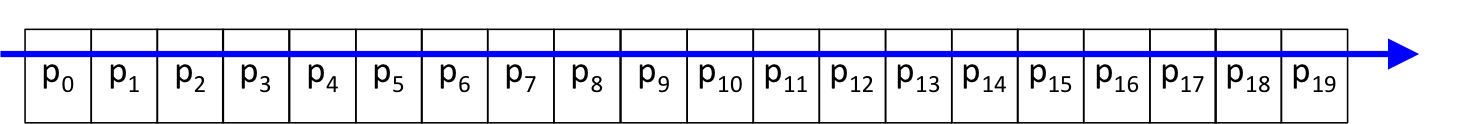### Multithreading with OpenMP

To parallelize our image-processing operation, we will use a library called OpenMP that is supported by most modern C/C++ compilers, including the GNU compiler suite (gcc/g++), the C/C++ compilers in MS-Visual Studio, and Intel's C/C++ compilers.

To see how multithreading works, suppose that we have a normal C/C++ method, and the flow of work it does (i.e., the sequence of statements it performs) is represented by a blue line. A traditional program might look like this, as it executes from start to finish: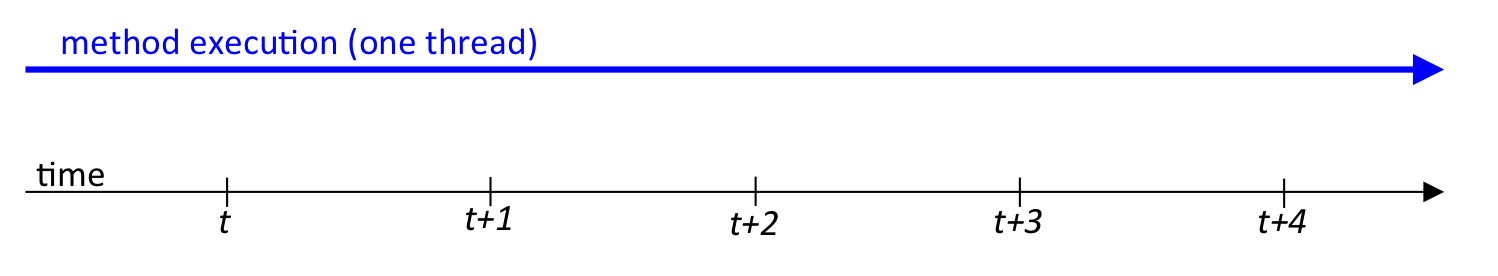Suppose further that the operations in the method between time t and time t+4 can be performed in parallel. When this is the case, a programmer can embed an OpenMP #pragma directive into his or her program to form a parallel region. When the program execution reaches one of these parallel regions, the program's single work-flow divides into multiple threads, normally one per core. So on a quad-core CPU, our picture above changes to one like this: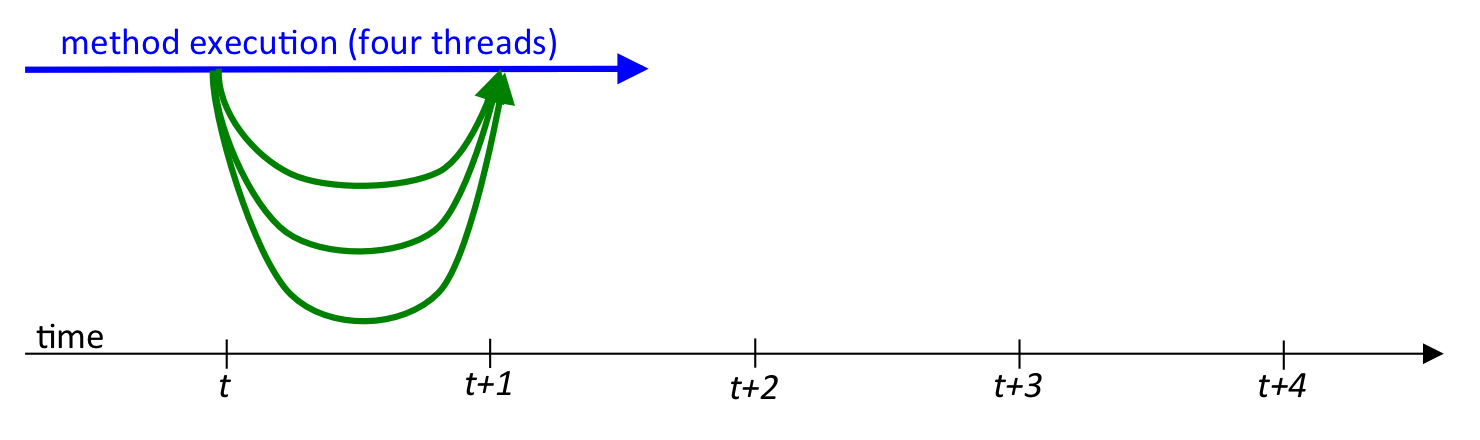In this diagram, the blue line represents the original "parent" or "master" thread, and the green lines represent the new "child" threads that OpenMP forms when the program flow reaches the parallel region. On a quad-core computer, each thread can run in parallel on a different core. If we structure our code so each of the four threads does about 1/4 of the work, then the total amount of work done during the computation remains the same, but because each thread does its piece of the computation in parallel with the others, the method will finish much faster than if a single thread was used.

### Multithreaded Image Processing

In today's exercise, we will be using this approach to "parallelize" an image-processing operation, so that it runs faster. To see how, let's return to our simple 4x5 image. As we saw earlier, a single-threaded program just processes the image's pixels, one at a time, in sequence:However, if we had two threads instead of one, we might divide up the work so that the parent thread processes the top half of the image and the child thread processes the bottom half: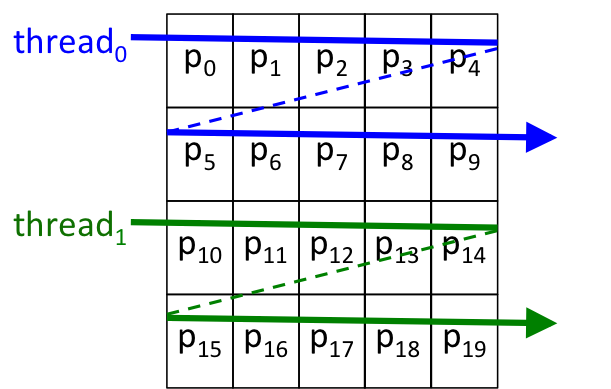Recalling that the image is stored in memory row-by-row, this approach will cause each of the two threads to process its own "chunk" of the pixels: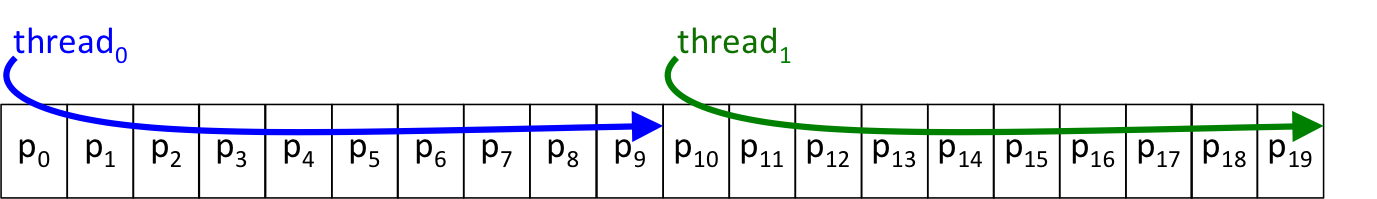What if we had four threads? It should be evident that then we could give each thread 1/4 of the image to process: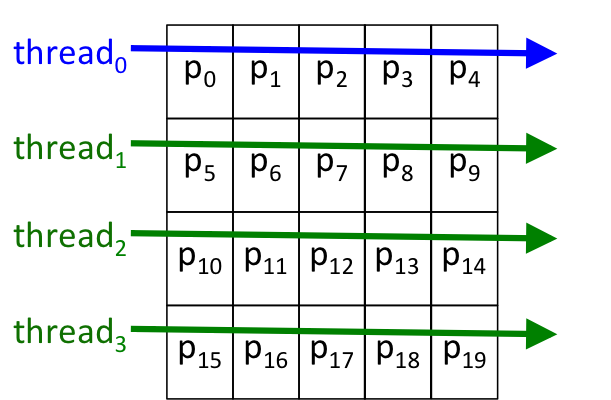in which case each thread would still get its own "chunk" of the pixels to process: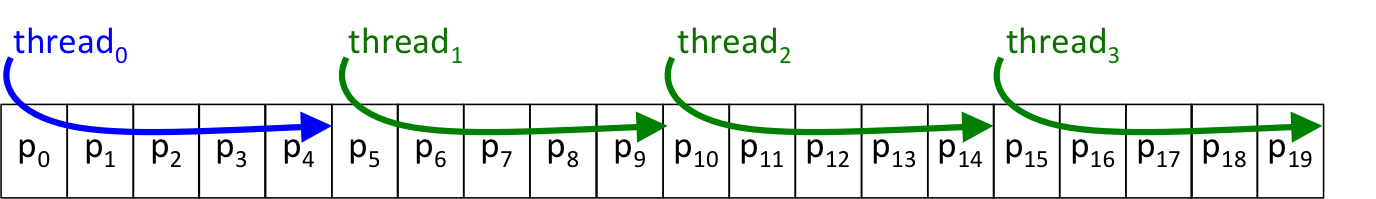The approach we will use today is:

2. Add timing calls to measure how long it takes.
3. Add OpenMP code to "parallelize" the method using multiple threads.
4. Measure how long the method takes using different numbers of threads.
5. Create a visualization of our results.

As you can see, an important part of today's exercise is timing the operation. The more applications you have running, the more competition your program will have for memory and the CPU, and the less accurate your timing results will be, so close down all non-essential applications (e.g., e-mail, chat clients, extra browser tabs, etc.) before proceeding.

### Getting Started

With that by way of introduction, let's get started.

In the open source world of Linux/Unix, the source code for a software project is often distributed as a tarball -- a folder of files that has been archived using the tar command, and then compressed to make it consume less space. Because we have several files for today's exercise, we have combined them into a single tarball for you to download, so that you don't have to download each file individually.

Our tarball is named ImageConverter.tgz, so begin by downloading it. Save it somewhere convenient (e.g., your desktop). The .tgz extension on this file indicates that it was tarred and then compressed with the GNU ZIP-compression utility gzip.

To open the tarball, launch a terminal, and use the ls and cd commands to navigate to the directory/folder where you saved the tarball.

When you are in right folder, enter this command:

```   tar xvzf ImageConverter.tgz
```
• The x switch tells tar to extract the tarred folder,
• the v tells it to do so verbosely (i.e., with lots of output),
• the z tells it that the tarball has been zipped (compressed) so it will need to be unzipped (decompressed), and
• the f tells it that the very next thing in the command is the name of the tarball file.
Thanks to the v switch, you should see something like this:
```   x ImageConverter/
x ImageConverter/pics/
x ImageConverter/pics/colorCrayons.jpg
x ImageConverter/pics/coloredMarkers.jpg
x ImageConverter/pics/colorfulCars.jpg
x ImageConverter/pics/redEye.jpg
x ImageConverter/ImageConverter.cpp
x ImageConverter/ImageConverter.h
x ImageConverter/main.cpp
x ImageConverter/Makefile
```
If you enter the ls command, you should now see both the ImageConverter.tgz tarball and the ImageConverter folder listed. If you enter:
```   ls ImageConverter
```
you should see these files listed:
```   ImageConverter.cpp
ImageConverter.h
Makefile
main.cpp
```
plus a folder named pics that contains some colorful image files.

Next, launch Eclipse, as usual, but when you use File > New > C++ Project to create a new project for today's exercise, specify

```    Makefile project > Empty Project
```
in the C++ Project dialog box, and make certain that the Linux GCC option is selected under Toolchains: before you click the Finish button. (This does not currently happen by default for a 'Makefile project', so be sure you select it manually.)

Once you have created the new project as a 'Makefile project', import all the files (using Select all in the Import dialog box) that you extracted from the tarball into your new project.

Then open main.cpp, ImageConverter.cpp, and ImageConverter.h, and take a few minutes to look over their contents, to see how they relate to one another. Once you understand what each file does, continue.

### Building

In the open source Linux/Unix world, a program called make is used to compile a tarball's source code into an application. To coordinate the compilation, a file named Makefile is usually included in the tarball. The tarball for this exercise includes a Makefile to simplify the task of building our multicore application, which is the reason we told Eclipse to create an empty Makefile project.

(Explaining how make works is beyond the scope of this exercise, but a Makefile is a text file, so feel free to open up the Makefile in Eclipse and examine its contents. If you are interested in learning more about how it works, here is a tutorial on make that will get you started.)

The make program reads this file and uses the relationships and commands on these lines to compile and link the files in the project.)

Given this Makefile in your project folder, you should be able to build and run your project from within Eclipse, as usual. When you build your program, Eclipse will execute

```   make all
```
in your project folder, the same as usual. This will invoke the make program, tell it to build the target all, and it will look for a file named Makefile that tells it how to do that. When make is finished building your program, you should be able to run it from within Eclipse, as usual.

It is worth mentioning that in a standard (i.e., non-Makefile) Eclipse project, there is still a Makefile behind the scenes that coordinates the compilation and linking. The difference is that in a standard project, Eclipse auto-generates its own Makefile (it is stored in the project's Debug folder), while in a Makefile-project, the programmer must supply their own Makefile. Since TSGL is a non-standard graphics library, we have supplied the Makefile to inform the C++ compiler (g++) of the location of tsgl.h and the TSGL binary library.

Take a moment to view the program in main.cpp. Note that it currently just creates an ImageConverter object:

```   #include "ImageConverter.h"

int main() {
ImageConverter iConverter("pics/beads.jpg", 800, 800);
//    iConverter.invertImage();
}
```
This call to the ImageConverter constructor creates an 800x800 Canvas object and loads an image from the specified file into that object. Build and run the program, and verify that it displays a Canvas object containing the following picture of beads of various colors:Click the "close box" on each Canvas object's frame (or press Escape), and then continue.

In the file ImageConverter.h, note that the ImageConverter class has two methods and four instance variables:

```   class ImageConverter {
public:
ImageConverter(const std::string& imageFileName, int width, int height);
~ImageConverter();

private:
Canvas      myCanvas1;
string      myImageFileName;
int         myWidth;
int         myHeight;
};
```
The two methods allow us to construct an ImageConverter object from a given image file, and clean up at the end of its lifetime. The four instance variables provide a Canvas in which the image will be displayed, a string in which the name of the image file is stored, and the desired width and height of the Canvas.

The Canvas class is declared in a graphics library developed at Calvin called TSGL, which stands for "thread safe graphics library". Unlike other graphical libraries, TSGL permits different threads to draw to the same graphical canvas at the same time, and displays the results in approximate real-time, which is ideal for today's exercise.

#### The ImageConverter Constructor

In the file ImageConverter.cpp, locate the ImageConverter constructor:

```   ImageConverter::ImageConverter(const std::string& imageFileName, int width, int height)
: myCanvas1(0, 0, width, height, imageFileName),
myImageFileName(imageFileName),
myWidth(width),
myHeight(height)
{
myCanvas1.start();
myCanvas1.drawImage(myImageFileName, 0, 0, width, height);
sleep(1);
}
```

As can be seen above, this constructor has 3 parameters: the name of the image file, and the desired width and height in which to display the image. Using these 3 parameters, the constructor initializes a Canvas object, and then saves the parameter values in the instance variables: myWidth, myHeight, and myImageFileName. All of this happens in the constructor's initialization-list area, which provides an alternative way of initializing a class's instance variables. The initializations in this initialization-list area are performed before the body of the constructor executes, so if the body depends on any of those initial values, this provides a means of ensuring that they have been stored.

The body of the constructor sends myCanvas1 the start() message, which activates that Canvas and makes it appear. It then sends myCanvas1 the drawImage() message, with the arguments that method requires to read and display the image from fileName. The drawImage() method uses a separate thread to load and display the image asynchronously from the main thread, so the final line of the constructor invokes the sleep() system call to put the main thread to sleep for a second, in order to give the second thread time to finish loading and displaying the image on myCanvas1 before continuing.

#### The ImageConverter Destructor

Next, find the definition of the ImageConverter destructor in ImageConverter.cpp:

```   ImageConverter::~ImageConverter() {
myCanvas1.wait();
cout << "\nImageConverter terminated normally." << endl;
}
```
In main.cpp, at the end of our ImageConverter object's scope, this destructor is invoked (invisibly). It sends the wait() message to the Canvas instance variable myCanvas1. This message causes myCanvas1 to pause until the user clicks the window's close box or presses the Escape key while the window has mouse-focus. Once the user has done that, the destructor displays a "normal termination" feedback message for the user.

The rules for using a TSGL Canvas include:

• A Canvas object should be sent the start() message before other graphical operations are performed on it.
• A Canvas object should be sent the wait() message when you are done drawing on it. This method causes the program to wait until the Canvas's "close box" is clicked or the user presses the Escape key.
The Canvas methods start() and wait() act like "bookends" for using a Canvas object.

### Image Inversion

Now that we have seen how the ImageConverter constructor and destructor work, let's proceed to our image inversion operation. The inverse of a color image (or the negative of a black and white image) is just the inverse of each of its pixels. That is, we can compute the inverse of an image by iterating through its pixels and computing the inverse of each pixel, using the following steps:

```    color = canvas0.getPixel(row,col);
invertedR = 255 - color.R;
invertedG = 255 - color.G;
invertedB = 255 - color.B;
invertedColor = RGB_Color(invertedR, invertedG, invertedB);
canvas1.setPixel(row, col, invertedColor);
```
To illustrate, these steps will convert a pixel that is colored:
• Black (0,0,0) to White (255,255,255).
• White (255,255,255) to Black (0,0,0).
• Green (0,255,0) to Magenta (255,0,255).
• Magenta (255,0,255) to Green (0,255,0).
• A dark gray (10,10,10) to a light gray (245,245,245), and vice versa.
• ...
In short, these steps convert a color to its opposite in the color palette, and the same steps can be used to convert that opposite color back to the original color.

#### Part A. Inverting an Image Sequentially

The invertImage() method shown below uses this approach; take a moment to copy-and-paste this definition into ImageConverter.cpp:
```   void ImageConverter::invertImage() {
Canvas canvas2(myWidth+50, 0, myWidth, myHeight, myImageFileName + " Inverted");
canvas2.start();

// loop through the image rows
for (int row = 0; row < myHeight; row++) {
// slow the processing to simulate a very large image
canvas2.sleep();
// loop through the image columns
for (int col = 0; col < myWidth; col++) {
// read the pixel at canvas1[row][col]
ColorInt pixelColor = myCanvas1.getPixel(row, col);
// compute its inverse
int invertedR = 255 - pixelColor.R;
int invertedG = 255 - pixelColor.G;
int invertedB = 255 - pixelColor.B;
// draw the inverse at the same spot in canvas2
canvas2.drawPixel(row, col, ColorInt(invertedR,invertedG,invertedB) );
} // inner for
} // outer for

canvas2.wait();
} // invertImage
```
You will also need to add a prototype for this method to the ImageConverter class in ImageConverter.h.

This method creates a new, local Canvas object named canvas2, and then enters a pair of nested for loops that, for each pixel in the image in myCanvas1, reads the pixel, computes its inverse, and then draws the inverted pixel on canvas2. Once it is done drawing, this second Canvas waits for the user to click the close-box or press Escape.

In TSGL, RGB colors are represented using the ColorInt type, as can be seen in the declaration of the pixelColor variable within the invertImage() method.

Testing invertImage(). Uncomment the call to invertImage() in main.cpp. Then save all changes, rebuild your program, run it, and verify that it correctly produces an inverted version of the beads.jpg image. Thanks to our use of TSGL, you should see the program's single thread drawing the inverted image from top to bottom.

Note that this image is an 1024x1024 (1 megapixel) image, but our main() function tells the ImageConverter to down-scale it to 800x800, in order to fit our screens. Unlike a larger (e.g., 120 megapixel) image, the processing of such a small image will happen too fast for us to see the thread drawing it, so our invertImage() method uses a sleep() call before each row is drawn, to slow the processing sufficiently for us to see the drawing. Using the Canvas::sleep() method this way lets us process an image that fits on our screen, but simulates how long it would take our program to process a much larger image.

Find the statement in invertImage() that constructs canvas2. Feel free to experiment with the arguments to see how changing them affects the construction of canvas2.

Timing invertImage(). To determine how long this operation is taking, modify invertImage() so that it appears as follows:

```   void ImageConverter::invertImage() {
// record starting time
double startTime = omp_get_wtime();

Canvas canvas2(myWidth+50, 0, myWidth, myHeight, myImageFileName + " Inverted");
canvas2.start();

// loop through the image rows
for (int row = 0; row < myHeight; row++) {
// slow the processing to simulate a very large image
canvas2.sleep();
// loop through the image columns
for (int col = 0; col < myWidth; col++) {
// read the pixel at canvas1[row][col]
ColorInt pixelColor = myCanvas1.getPixel(row, col);
// compute its inverse
int invertedR = 255 - pixelColor.R;
int invertedG = 255 - pixelColor.G;
int invertedB = 255 - pixelColor.B;
// draw the inverse at the same spot in canvas2
canvas2.drawPixel(row, col, ColorInt(invertedR,invertedG,invertedB) );
} // inner for
} // outer for

// compute and display the time difference
double totalTime = omp_get_wtime() - startTime;
cout << "\n\nImage inversion took "
<< totalTime << " seconds.\n" << endl;

canvas2.wait();
} // invertImage
```
The omp_get_wtime() function returns the number of seconds that have elapsed since the program began running. By calling and storing its value before the nested loops, and then calling it again after the nested loops, we can compute the difference in the two times, which will be the time our program has spent inverting the image.

With that change made, rebuild and rerun your program. The time required to perform this operation should now appear in the Eclipse console. Compare the value you see with one of your neighbors; your times should be fairly close to one another.

Using your favorite spreadsheet program, open up a worksheet. On its first row, place a descriptive title (e.g., CS 112 Lab 10: Image Inversion, ...) Be sure to include your name and the date.

Beneath that, make two column-headings: one labeled NumThreads and one labeled Time. Since we used 1 thread just now, enter a 1 under NumThreads; under Time, enter the time from the Eclipse console.

#### Part B. Parallel Image Inversion Using "#pragma omp parallel for"

Now that we have recorded the time required to invert the image sequentially, we are ready to "parallelize" this operation. We will do this in two steps:

1. Modifying the main() function so that we can specify how many threads to use; and
2. Modifying the invertImage() method to spread the work performed by the outer loop across multiple threads.

1. Modifying main(). There are several ways to control the number of threads OpenMP uses for parallel processing:

• By defining an environment variable OMP_NUM_THREADS and setting it to the desired number.
• By using a num_threads(n) clause with OpenMP directives, where n is the desired number of threads.
• By using the OpenMP function omp_set_num_threads(n); to set the desired number of threads to n.
We will use the last approach, so in main.cpp, modify the main() function so that it appears as follows:
```   #include "ImageConverter.h"

int main() {

ImageConverter iConverter("pics/beads.jpg", 8o0, 800);
iConverter.invertImage();
}
```
We will start with 1 thread, to ensure that our modifications will not "break" our program if a single thread is used.

However, by changing the argument to omp_set_num_threads(), we can easily control the number of threads OpenMP will use. That will let us see how varying the number of threads affects the time required to process our image.

2. Modifying invertImage(). If a loop's iterations are independent of one another, OpenMP makes it easy to spread the iterations of that loop across different threads.

Modify the body of invertImage() by inserting the #pragma directive shown below:

```   void ImageConverter::invertImage() {
// record starting time
double startTime = omp_get_wtime();

Canvas canvas2(myWidth+50, 0, myWidth, myHeight, myImageFileName + " Inverted");
canvas2.start();

// loop through the image rows
#pragma omp parallel for
for (int row = 0; row < myHeight; row++) {
// slow the processing to simulate a very large image
canvas2.sleep();
// loop through the image columns
for (int col = 0; col < myWidth; col++) {
// read the pixel at canvas1[row][col]
ColorInt pixelColor = myCanvas1.getPixel(row, col);
// compute its inverse
int invertedR = 255 - pixelColor.R;
int invertedG = 255 - pixelColor.G;
int invertedB = 255 - pixelColor.B;
// draw the inverse at the same spot in canvas2
canvas2.drawPixel(row, col, ColorInt(invertedR,invertedG,invertedB) );
} // inner for
} // outer for

// compute and display the time difference
double totalTime = omp_get_wtime() - startTime;
cout << "\n\nImage inversion took "
<< totalTime << " seconds.\n" << endl;

canvas2.wait();
} // invertImage
```
A #pragma directive is a suggestion to the C/C++ compiler. In the case of our directive:
```   #pragma omp parallel for
```
if the compiler does not support OpenMP, then it will ignore this suggestion but still compile your program. However, if the compiler does support OpenMP, then the directive is a very strong suggestion to the compiler that:
1. When this statement is reached, it should spawn additional threads, with the number of threads specified by: (i) a num_threads(n) clause, if present; (ii) the most recent call to omp_set_num_threads(n); or (iii) the value of the environment variable OMP_NUM_THREADS, which typically defaults to the number of cores on your computer.
2.
3. In the for loop that follows the directive, the iterations of that loop be divided among the n threads as evenly as possible. If the number of iterations is evenly divisible by n:
• Thread 0 perform the first 1/n of the loop's iterations.
• Thread 1 perform the next 1/n of the loop's iterations.
• ...
• Thread n-1 perform the final 1/n of the loop's iterations.
To illustrate, the outer loop of our invertImage() method is currently processing an image with 800 rows. With 2 threads, this directive will divide that loop's 800 iterations between thread 0 and thread 1, with thread 0 performing the first half of the iterations (i.e., in which its loop-variable row gets the values 0-399) and thread 1 performing the remaining half of the iterations (i.e., in which its loop-variable row gets the values 400-799). Since the loop-variable row determines the number of the image-row being processed, the effect is that thread 0 will process the top half of the image and thread 1 will process the bottom half of the image.

Note that in order for this to work, OpenMP must give each thread its own private copy of the loop-variable row, or else the two threads may conflict with one another when their loops increment row. In fact, OpenMP gives each thread its own copy of any variable declared within the loop. By contrast, any variable declared before the #pragma directive is shared among the threads, which can cause conflicts.

Testing. Having made these changes, rebuild and rerun your program. You should still see a single thread processing the image from top to bottom, because we only told OpenMP to use 1 thread in its parallel regions. Verify that the resulting image is still correctly inverted before continuing.

Timing. Back in main.cpp, change the argument to omp_set_num_threads() from 1 to 2. The rebuild and rerun your program. Instead of a single thread, you should now see two threads working in tandem, each processing one half of the image in a top-down fashion.

When the threads have finished, the time they took to process the image should appear in your Eclipse console, as before. (This should be about 1/2 the time required by the sequential (single-threaded) approach.) Record this number of threads and the time in your spreadsheet.

Varying the Number of Threads. Next, we want to see how long it takes to process our image using 4, 6, 8, 10, 12, 14, and 16 threads.

In main.cpp, change the argument to omp_set_num_threads() from 2 to 4. Then rebuild and rerun your program. You should now see four threads working and processing their quarters of the image in parallel. When they have finished, record the number of threads and the time in your spreadsheet. (This should be about 1/4 the time of the sequential approach.)

Then repeat this procedure for 6, 8, 10, 12, 14, and 16 threads, using the spreadsheet to record the processing time for each the number of threads.

Visualizing the Improvement. In your spreadsheet, create a line-chart that shows the relationship between the number of threads being used and the time required to invert the image. Put Time on the Y-axis and Number of Threads on the X-axis. Be sure to label your chart and axes (including units); here is one I created using Google's spreadsheet program: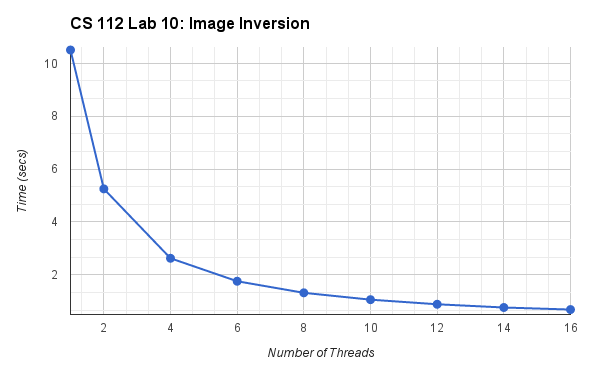Saving the Inverted Image. Saving an image isn't necessary for parallel execution. But if we have gone to the trouble of creating a cool transformation of an image, we may want to save the result. One way to do this is using TSGL's Canvas::takeScreenShot() method. When sent to a Canvas object, this message dumps a portable-network-graphics-formatted (.png) copy of the Canvas into the current folder/directory. We can thus save the result of our conversion by tweaking the invertImage() method as follows:

```   void ImageConverter::invertImage() {
// record starting time
double startTime = omp_get_wtime();

Canvas canvas2(myWidth+50, 0, myWidth, myHeight, myImageFileName + " Inverted");
canvas2.start();

// loop through the image rows
#pragma omp parallel for
for (int row = 0; row < myHeight; row++) {
// slow the processing to simulate a very large image
canvas2.sleep();
// loop through the image columns
for (int col = 0; col < myWidth; col++) {
// read the pixel at canvas1[row][col]
ColorInt pixelColor = myCanvas1.getPixel(row, col);
// compute its inverse
int invertedR = 255 - pixelColor.R;
int invertedG = 255 - pixelColor.G;
int invertedB = 255 - pixelColor.B;
// draw the inverse at the same spot in canvas2
canvas2.drawPixel(row, col, ColorInt(invertedR,invertedG,invertedB) );
} // inner for
} // outer for

// compute and display the time difference
double totalTime = omp_get_wtime() - startTime;
cout << "\n\nImage inversion took "
<< totalTime << " seconds.\n" << endl;

//save converted image
canvas2.takeScreenShot();
canvas2.wait();
} // invertImage
```
Make this change. Then rebuild and rerun your program. A file with a cryptic name like Image000079.bmp should appear in your project folder. (You may have to rightclick > Refresh your project folder -- or press F5 -- to see it.) You can view this image from with Eclipse by using rightClick > Open with > Other... > Internal Web Browser, or from within your regular web browser by choosing File > Open file... and navigating to your project folder and selecting this file.

Using either approach, verify that this bitmap file contains a copy of the converted image, before continuing.

How Does OpenMP Do It?.

We just saw that OpenMP makes it easy to "parallelize" the iterations of a loop. However in making it so easy, it hides most of the details as to how it accomplishes this. There are different ways that a loop's iterations can be "parallelized", including:

1. A simpler, "chunk-size 1" approach; and
2. A less simple "equal-sized chunks" approach.
In the rest of today's exercise, we will explore these two approaches, to try and see which one OpenMP uses.

Parallel Blocks.

OpenMP has a #pragma omp parallel directive that is simpler and more general than the #pragma omp parallel for directive. Using four threads, it behaves as follows: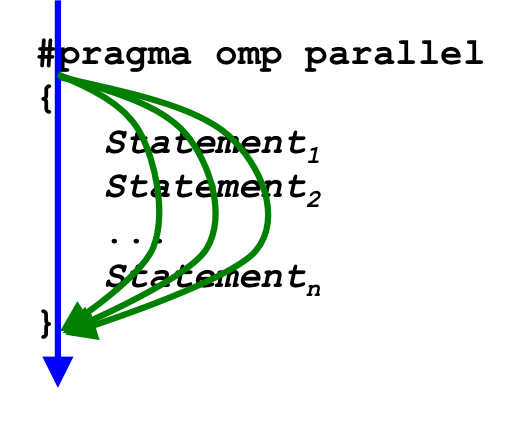That is, when the flow of execution reaches a #pragma omp parallel directive, it launches additional threads (as described previously), and each thread performs the block of statements that follow the directive. For this reason, this combination of directive and block is sometimes called a parallel block.

(The #pragma omp parallel for directive that we used previously is a special case of the more general #pragma omp parallel directive. When the for is present in the pragma, the next statement following the directive must be a for loop, instead of a block.)

Using a parallel block, we can implement either approach to loop parallelization.

#### Part C. Parallel Processing Using The "Chunk Size 1" Approach

The simpler "chunk-size 1" approach is shown below.

```
void ImageConverter::invertImage2() {

Canvas canvas3(myWidth+50, 0, myWidth, myHeight, myImageFileName + " Inverted, Chunk-Size 1");
canvas3.start();

#pragma omp parallel
{
// how many workers do we have?
// which one am I?
unsigned id = omp_get_thread_num();

// loop through the image rows
for (int row = id; row < myHeight; row += numThreads) {
// slow the processing to simulate a very large image
canvas3.sleep();
// loop through the image columns
for (int col = 0; col < myWidth; col++) {
// read the pixel at canvas1[row][col]
ColorInt pixelColor = myCanvas1.getPixel(row, col);
// compute its inverse
int invertedR = 255 - pixelColor.R;
int invertedG = 255 - pixelColor.G;
int invertedB = 255 - pixelColor.B;
// draw the inverse at the same spot in canvas2
canvas3.drawPixel(row, col, ColorInt(invertedR,invertedG,invertedB) );
} // inner for
} // outer for
} // #pragma omp parallel

canvas3.wait();
} // invertImage2
```
Take a moment to copy this definition into ImageConverter.cpp, below the definition of invertImage(). You will also need to declare a prototype for this method in the ImageConverter class.

How It Works. To see how this approach works, suppose that main.cpp contains omp_set_num_threads(4), so the #pragma launches four threads.

1. Each thread performs the statement:
`      unsigned numThreads = omp_get_num_threads(); `
For each thread, the omp_get_num_threads() function returns the same value (4), so following this, numThreads == 4 for all four threads.

2. Each thread performs the statement:
`      unsigned id = omp_get_thread_num(); `
When each thread performs this statement, the omp_get_thread_num() returns a different value, namely the id number for that thread. In the parent thread, the function returns 0; in the first child thread, the function returns 1; in the second child thread, the function returns 2; and in the final child thread, the function returns 3. Using n threads, the omp_get_thread_num() function will return n different values, ranging from 0 to n-1. So following this statement, the variable id will have a different value in each thread.

3. Each thread enters the outer for loop:
`      for (unsigned row = id; row < myHeight; row += numThreads) `
Since each thread has a different value in variable id, each thread starts at a different row of the image. And since each adds numThreads (e.g., 4) to variable row each loop iteration:
• Thread 0 processes rows 0, 4, 8, 16, ..., 836.
• Thread 1 processes rows 1, 5, 9, 17, ..., 837.
• Thread 2 processes rows 2, 6, 10, 18, ..., 838.
• Thread 3 processes rows 3, 7, 11, 19, ..., 839.
All rows thus get processed, as each of the n threads starts at a different row, and when it is finished with that row, skips forward n rows and processes that row.
Put differently, this approach divides the loop's interations into "chunks", but each chunk consists of a single integer value. Each thread thus gets many chunks to process, each of size 1.

Testing. In main.cpp, add a call to invertImage2(), below the call to invertImage(). Then rebuild and run the program, and compare the behavior produced by the two methods.

Since the number of available cores varies from computer to computer, it is important for parallel operations to run correctly regardless of the number of available cores. On the software side, the operation needs to produce correct results regardless of whether one or many threads are being used.

Try this approach using 1, 2, 4, 6, 8, 10, 12, 14, and 16 threads, and verify that it correctly processes the image for each value. Then answer the following question in your spreadsheet:

1. Does the #pragma omp parallel for directive divide up a loop's iterations the same way as the "chunk-size 1" approach? Explain your answer.

#### Part D. Parallel Processing Using the "Equal-Sized Chunks" Approach

Now that we have compared the #pragma omp parallel for approach and the "Chunk-Size 1" approach, let's examine a different approach we will call "Equal-Sized Chunks". This approach divides the loop's iterations into equal-sized chunks, and gives each thread one of those chunks to process. One way to do this is shown below:

```
void ImageConverter::invertImage3() {

Canvas canvas4(myWidth+50, 0, myWidth, myHeight, myImageFileName + " Inverted, Equal-Sized Chunks ");
canvas4.start();

#pragma omp parallel
{
// how many workers do we have?
// which one am I?
unsigned id = omp_get_thread_num();

// compute size of chunks (iterations % numThreads may != 0)
double iterations = myHeight;
unsigned chunkSize = (unsigned)ceil(iterations / numThreads);

// compute starting and stopping index values
int start = id * chunkSize;
int stop = 0;
if (id < numThreads-1) {
stop = start + chunkSize;
} else {
stop = myHeight;
}

// loop through image rows in equal-sized chunks
for (int row = start; row < stop; row++) {
// slow the processing to simulate a very large image
canvas4.sleep();
// loop through the image columns
for (int col = 0; col < myWidth; col++) {
// read the pixel at canvas1[row][col]
ColorInt pixelColor = myCanvas1.getPixel(row, col);
// compute its inverse
int invertedR = 255 - pixelColor.R;
int invertedG = 255 - pixelColor.G;
int invertedB = 255 - pixelColor.B;
// draw the inverse at the same spot in canvas2
canvas4.drawPixel(row, col, ColorInt(invertedR,invertedG,invertedB) );
} // inner for
} // outer for
} // #pragma omp parallel

canvas4.wait();
} // invertImage3
```
Take a moment to copy this definition into ImageConverter.cpp, and add its prototype to the ImageConverter class. You may also need to #include <cmath> in order for the ceil() function to be defined.

How It Works. To see how this approach works:

1. When execution reaches the #pragma omp parallel directive, OpenMP launches additional threads as we saw before. For example, if we have four threads processing our image of 800 rows, each thread again computes a common numThreads value (4), and its own id value (0, 1, 2, or 3).

2. Each thread then computes a common value for the chunkSize by dividing the number of iterations by the number of threads. Since the number of iterations may not be evenly divisible by the number of threads, we use the ceiling function ceil() to round the resulting quotient up, if necessary:
```      double iterations = myHeight;
unsigned chunkSize = (unsigned)ceil(iterations / numThreads); ```
In our case, iterations is the number of rows in the image (800), so each thread computes 800 / 4 = 200 as the value of chunkSize.

3. Each thread then uses its id and the chunkSize to compute its unique starting index:
`      unsigned start = id * chunkSize; `
In our four-thread scenario where chunkSize is 200:
• Thread 0 will compute 0 x 200 = 0 for its start value.
• Thread 1 will compute 1 x 200 = 200 for its start value.
• Thread 2 will compute 2 x 200 = 400 for its start value.
• Thread 3 will compute 3 x 200 = 600 for its start value.
Unlike the "chunk-size 1" approach in which consecutive-numbered threads start on consecutive-numbered rows, this approach has each thread start its processing on a row that is chunkSize (200) rows away from the other threads.

4. Each thread then computes its unique stopping index:
```     unsigned stop = 0;
if (id < numThreads-1) {
stop = start + chunkSize;
} else {
stop = myHeight;
} ```
In this step, all threads except the final thread stop processing when they reach start of the next thread's chunk. Thus:
• Thread 0 will compute 0 + 200 = 200 for its stop value.
• Thread 1 will compute 200 + 200 = 400 for its stop value.
• Thread 2 will compute 400 + 200 = 600 for its stop value.
• Thread 3 is the final thread, so its stop value will be set to myHeight (800).

(If the number of iterations is not evenly divisible by the number of threads, then this if statement keeps the final thread from going out of bounds. For example, if our image had 799 rows instead of 800, the if statement would ensure that thread 3 stops after it finishes processing row 798.)

5. With start and stop values computed for each thread, we are ready to divide up the iterations of our loop:
`      for (unsigned row = start; row < stop; row++) { `
As can be seen, each thread starts processing the row at its start index value, and iterates through consecutive rows until it reaches its stop index value.
This "equal-sized chunks" approach thus divides a loop's iterations so that each thread processes "chunks" of consecutive iterations that are equal in size (unless the number iterations is not evenly divisible by the number of threads, in which case the final thread may have a slightly smaller chunk).

Add a call to invertImage3() in main() below the calls to invertImage() and invertImage2().

Testing and Visualization. Rebuild and run your program, using 1, 2, 4, 6, 8, 10, 12, 14, and 16 threads, and verify that it correctly processes the image for each value. Then answer the following question in your spreadsheet:

1. Does OpenMP's #pragma omp parallel for directive use either of these two approaches ("chunk-size 1" vs. "equal-sized chunks") to divide up the loop's iterations? Explain your answer.
2.
3. We have only examined one image processing operation, but on the basis of what you have observed, what seems to be the relationship between the number of threads used to process an image and the time required to complete the image-processing operation?

Other Images. There are other image files in the pics folder that you downloaded with the tarball. As time permits, feel free to experiment with some of these other images to verify that your operations work on them, too. (The file colorfulCars.jpg has especially nice colors.)

### Wrapping Up

In image processing methods like inversion, each thread is accessing a group of pixels that is distinct from the pixels being accessed by the other threads.

For example, if we process an image using invertImage() and two threads, thread 0 accesses the top half of the image, and thread 1 accesses the bottom half of the image. Since there is no overlap in the pixels each thread is accessing. each thread can process its part of the image without any interference from other threads.

Problems like this -- where the nature of the problem is such that each thread can solve its piece of the problem without having to interact with other threads -- are called embarrassingly parallel problems. Not that there is anything embarrassing about the problems; these are just the easiest kind of problem to solve in parallel -- so easy that it would be embarrassing to still solve them sequentially, once we know about parallelism!

Not all problems are embarrassingly parallel. A problem might require one thread to read a variable's value at the same time as another thread might be writing to that same variable, or for two threads to write to the same variable. In problems like this, the accesses to those data values must be synchronized, or the results are non-deterministic (i.e., unpredictable). This is generally a Bad Thing for a computer program.

Computer scientists call unsynchronized accesses to common data values race conditions or data races, because the threads can be thought of as "racing" to access the shared data values, and the computation's result depends on which thread wins/loses the race. Dealing with race conditions generally makes parallel programming more challenging than what we have experienced today.

Image processing is an ideal first problem for learning about parallelization, because it lets us focus on the parallel concepts without the complications of race conditions. However many (most?) problems are not so easily solved, so don't leave today's exercise with the impression that parallelization is always this easy!

### Turn In

Make sure your spreadsheet is saved in your project folder, in an Excel-compatible format (.xls). I recommend the one for 97/2000/XP, as it seems to work well.

Then copy your project to a new /home/cs/112/current/yourUserName/lab10 folder.

If you have time, you may wish to read over and begin work on this week's project, which is the final project for this course.

CS > 112 > Labs > 10